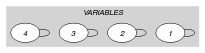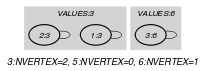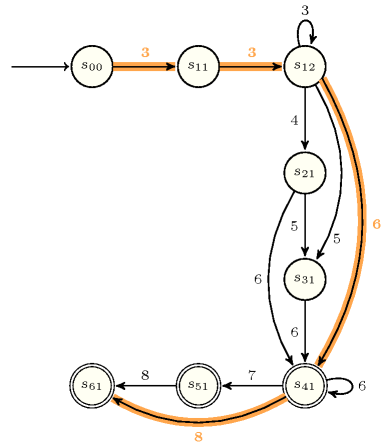## 5.186. increasing_global_cardinality

Origin
Constraint

$\mathrm{𝚒𝚗𝚌𝚛𝚎𝚊𝚜𝚒𝚗𝚐}_\mathrm{𝚐𝚕𝚘𝚋𝚊𝚕}_\mathrm{𝚌𝚊𝚛𝚍𝚒𝚗𝚊𝚕𝚒𝚝𝚢}\left(\mathrm{𝚅𝙰𝚁𝙸𝙰𝙱𝙻𝙴𝚂},\mathrm{𝚅𝙰𝙻𝚄𝙴𝚂}\right)$

Synonyms

$\mathrm{𝚒𝚗𝚌𝚛𝚎𝚊𝚜𝚒𝚗𝚐}_\mathrm{𝚐𝚕𝚘𝚋𝚊𝚕}_\mathrm{𝚌𝚊𝚛𝚍𝚒𝚗𝚊𝚕𝚒𝚝𝚢}_\mathrm{𝚕𝚘𝚠}_\mathrm{𝚞𝚙}$, $\mathrm{𝚒𝚗𝚌𝚛𝚎𝚊𝚜𝚒𝚗𝚐}_\mathrm{𝚐𝚌𝚌}$, $\mathrm{𝚒𝚗𝚌𝚛𝚎𝚊𝚜𝚒𝚗𝚐}_\mathrm{𝚐𝚌𝚌}_\mathrm{𝚕𝚘𝚠}_\mathrm{𝚞𝚙}$.

Arguments
 $\mathrm{𝚅𝙰𝚁𝙸𝙰𝙱𝙻𝙴𝚂}$ $\mathrm{𝚌𝚘𝚕𝚕𝚎𝚌𝚝𝚒𝚘𝚗}\left(\mathrm{𝚟𝚊𝚛}-\mathrm{𝚍𝚟𝚊𝚛}\right)$ $\mathrm{𝚅𝙰𝙻𝚄𝙴𝚂}$ $\mathrm{𝚌𝚘𝚕𝚕𝚎𝚌𝚝𝚒𝚘𝚗}\left(\mathrm{𝚟𝚊𝚕}-\mathrm{𝚒𝚗𝚝},\mathrm{𝚘𝚖𝚒𝚗}-\mathrm{𝚒𝚗𝚝},\mathrm{𝚘𝚖𝚊𝚡}-\mathrm{𝚒𝚗𝚝}\right)$
Restrictions
 $\mathrm{𝚛𝚎𝚚𝚞𝚒𝚛𝚎𝚍}$$\left(\mathrm{𝚅𝙰𝚁𝙸𝙰𝙱𝙻𝙴𝚂},\mathrm{𝚟𝚊𝚛}\right)$ $\mathrm{𝚒𝚗𝚌𝚛𝚎𝚊𝚜𝚒𝚗𝚐}$$\left(\mathrm{𝚅𝙰𝚁𝙸𝙰𝙱𝙻𝙴𝚂}\right)$ $|\mathrm{𝚅𝙰𝙻𝚄𝙴𝚂}|>0$ $\mathrm{𝚛𝚎𝚚𝚞𝚒𝚛𝚎𝚍}$$\left(\mathrm{𝚅𝙰𝙻𝚄𝙴𝚂},\left[\mathrm{𝚟𝚊𝚕},\mathrm{𝚘𝚖𝚒𝚗},\mathrm{𝚘𝚖𝚊𝚡}\right]\right)$ $\mathrm{𝚍𝚒𝚜𝚝𝚒𝚗𝚌𝚝}$$\left(\mathrm{𝚅𝙰𝙻𝚄𝙴𝚂},\mathrm{𝚟𝚊𝚕}\right)$ $\mathrm{𝚅𝙰𝙻𝚄𝙴𝚂}.\mathrm{𝚘𝚖𝚒𝚗}\ge 0$ $\mathrm{𝚅𝙰𝙻𝚄𝙴𝚂}.\mathrm{𝚘𝚖𝚊𝚡}\le |\mathrm{𝚅𝙰𝚁𝙸𝙰𝙱𝙻𝙴𝚂}|$ $\mathrm{𝚅𝙰𝙻𝚄𝙴𝚂}.\mathrm{𝚘𝚖𝚒𝚗}\le \mathrm{𝚅𝙰𝙻𝚄𝙴𝚂}.\mathrm{𝚘𝚖𝚊𝚡}$
Purpose

The variables of the collection $\mathrm{𝚅𝙰𝚁𝙸𝙰𝙱𝙻𝙴𝚂}$ are increasing. In addition, each value $\mathrm{𝚅𝙰𝙻𝚄𝙴𝚂}\left[i\right].\mathrm{𝚟𝚊𝚕}$ $\left(1\le i\le |\mathrm{𝚅𝙰𝙻𝚄𝙴𝚂}|\right)$ should be taken by at least $\mathrm{𝚅𝙰𝙻𝚄𝙴𝚂}\left[i\right].\mathrm{𝚘𝚖𝚒𝚗}$ and at most $\mathrm{𝚅𝙰𝙻𝚄𝙴𝚂}\left[i\right].\mathrm{𝚘𝚖𝚊𝚡}$ variables of the $\mathrm{𝚅𝙰𝚁𝙸𝙰𝙱𝙻𝙴𝚂}$ collection.

Example
$\left(\begin{array}{c}〈3,3,6,8〉,\hfill \\ 〈\begin{array}{ccc}\mathrm{𝚟𝚊𝚕}-3\hfill & \mathrm{𝚘𝚖𝚒𝚗}-2\hfill & \mathrm{𝚘𝚖𝚊𝚡}-3,\hfill \\ \mathrm{𝚟𝚊𝚕}-5\hfill & \mathrm{𝚘𝚖𝚒𝚗}-0\hfill & \mathrm{𝚘𝚖𝚊𝚡}-1,\hfill \\ \mathrm{𝚟𝚊𝚕}-6\hfill & \mathrm{𝚘𝚖𝚒𝚗}-1\hfill & \mathrm{𝚘𝚖𝚊𝚡}-2\hfill \end{array}〉\hfill \end{array}\right)$

The $\mathrm{𝚒𝚗𝚌𝚛𝚎𝚊𝚜𝚒𝚗𝚐}_\mathrm{𝚐𝚕𝚘𝚋𝚊𝚕}_\mathrm{𝚌𝚊𝚛𝚍𝚒𝚗𝚊𝚕𝚒𝚝𝚢}$ constraint holds since:

• The values of the collection $〈3,3,6,8〉$ are sorted in increasing order.

• Values 3, 5 and 6 are respectively used 2 ($2\le 2\le 3$), 0 ($0\le 0\le 1$) and 1 ($1\le 1\le 2$) times within the collection $〈3,3,6,8〉$ and since no constraint was specified for value 8.

Typical
 $|\mathrm{𝚅𝙰𝚁𝙸𝙰𝙱𝙻𝙴𝚂}|>1$ $\mathrm{𝚛𝚊𝚗𝚐𝚎}$$\left(\mathrm{𝚅𝙰𝚁𝙸𝙰𝙱𝙻𝙴𝚂}.\mathrm{𝚟𝚊𝚛}\right)>1$ $|\mathrm{𝚅𝙰𝙻𝚄𝙴𝚂}|>1$ $\mathrm{𝚅𝙰𝙻𝚄𝙴𝚂}.\mathrm{𝚘𝚖𝚒𝚗}\le |\mathrm{𝚅𝙰𝚁𝙸𝙰𝙱𝙻𝙴𝚂}|$ $\mathrm{𝚅𝙰𝙻𝚄𝙴𝚂}.\mathrm{𝚘𝚖𝚊𝚡}>0$ $\mathrm{𝚅𝙰𝙻𝚄𝙴𝚂}.\mathrm{𝚘𝚖𝚊𝚡}\le |\mathrm{𝚅𝙰𝚁𝙸𝙰𝙱𝙻𝙴𝚂}|$ $|\mathrm{𝚅𝙰𝚁𝙸𝙰𝙱𝙻𝙴𝚂}|>|\mathrm{𝚅𝙰𝙻𝚄𝙴𝚂}|$
Symmetry

Items of $\mathrm{𝚅𝙰𝙻𝚄𝙴𝚂}$ are permutable.

Usage

This constraint can be used in order to break symmetry in the context of the following pattern. We have a matrix $ℳ$ of variables with the same constraint on each row and a $\mathrm{𝚐𝚕𝚘𝚋𝚊𝚕}_\mathrm{𝚌𝚊𝚛𝚍𝚒𝚗𝚊𝚕𝚒𝚝𝚢}_\mathrm{𝚕𝚘𝚠}_\mathrm{𝚞𝚙}$ constraint on each column. Beside lexicographically ordering the rows of $ℳ$ with a $\mathrm{𝚕𝚎𝚡}_\mathrm{𝚌𝚑𝚊𝚒𝚗}_\mathrm{𝚕𝚎𝚜𝚜𝚎𝚚}$ constraint, one can also state a $\mathrm{𝚒𝚗𝚌𝚛𝚎𝚊𝚜𝚒𝚗𝚐}_\mathrm{𝚐𝚕𝚘𝚋𝚊𝚕}_\mathrm{𝚌𝚊𝚛𝚍𝚒𝚗𝚊𝚕𝚒𝚝𝚢}$ on the first column of $ℳ$ in order to improve propagation on the corresponding variables.

Reformulation

The $\mathrm{𝚒𝚗𝚌𝚛𝚎𝚊𝚜𝚒𝚗𝚐}_\mathrm{𝚐𝚕𝚘𝚋𝚊𝚕}_\mathrm{𝚌𝚊𝚛𝚍𝚒𝚗𝚊𝚕𝚒𝚝𝚢}$ constraint can be expressed in term of a conjunction of a $\mathrm{𝚐𝚕𝚘𝚋𝚊𝚕}_\mathrm{𝚌𝚊𝚛𝚍𝚒𝚗𝚊𝚕𝚒𝚝𝚢}_\mathrm{𝚕𝚘𝚠}_\mathrm{𝚞𝚙}$ and an $\mathrm{𝚒𝚗𝚌𝚛𝚎𝚊𝚜𝚒𝚗𝚐}$ constraints. Even if we achieve arc-consistency on these two constraints this hinders propagation as shown by the following small example.

We have two variables $X$ and $Y$ $\left(X\le Y\right)$, which both take their values in the set $\left\{2,3\right\}$. In addition, assume that the minimum number of occurrences of values 0, 1 and 2 are respectively equal to 0, 1 and 1. Similarly assume that, the maximum number of occurrences of values 0, 1 and 2 are respectively equal to 1, 1 and 2. The reformulation does not reduce the domain of variables $X$, $Y$ in any way, while the automaton described in the Automaton slot fixes $X$ to 2 and $Y$ to 3.

Keywords

For all items of $\mathrm{𝚅𝙰𝙻𝚄𝙴𝚂}$:

Arc input(s)

$\mathrm{𝚅𝙰𝚁𝙸𝙰𝙱𝙻𝙴𝚂}$

Arc generator
$\mathrm{𝑆𝐸𝐿𝐹}$$↦\mathrm{𝚌𝚘𝚕𝚕𝚎𝚌𝚝𝚒𝚘𝚗}\left(\mathrm{𝚟𝚊𝚛𝚒𝚊𝚋𝚕𝚎𝚜}\right)$

Arc arity
Arc constraint(s)
$\mathrm{𝚟𝚊𝚛𝚒𝚊𝚋𝚕𝚎𝚜}.\mathrm{𝚟𝚊𝚛}=\mathrm{𝚅𝙰𝙻𝚄𝙴𝚂}.\mathrm{𝚟𝚊𝚕}$
Graph property(ies)
 $•$$\mathrm{𝐍𝐕𝐄𝐑𝐓𝐄𝐗}$$\ge \mathrm{𝚅𝙰𝙻𝚄𝙴𝚂}.\mathrm{𝚘𝚖𝚒𝚗}$ $•$$\mathrm{𝐍𝐕𝐄𝐑𝐓𝐄𝐗}$$\le \mathrm{𝚅𝙰𝙻𝚄𝙴𝚂}.\mathrm{𝚘𝚖𝚊𝚡}$

Graph model

Since we want to express one unary constraint for each value we use the “For all items of $\mathrm{𝚅𝙰𝙻𝚄𝙴𝚂}$” iterator. Part (A) of Figure 5.186.1 shows the initial graphs associated with each value 3, 5 and 6 of the $\mathrm{𝚅𝙰𝙻𝚄𝙴𝚂}$ collection of the Example slot. Part (B) of Figure 5.186.1 shows the two corresponding final graphs respectively associated with values 3 and 6 that are both assigned to the variables of the $\mathrm{𝚅𝙰𝚁𝙸𝙰𝙱𝙻𝙴𝚂}$ collection (since value 5 is not assigned to any variable of the $\mathrm{𝚅𝙰𝚁𝙸𝙰𝙱𝙻𝙴𝚂}$ collection the final graph associated with value 5 is empty). Since we use the $\mathrm{𝐍𝐕𝐄𝐑𝐓𝐄𝐗}$ graph property, the vertices of the final graphs are stressed in bold.

##### Figure 5.186.1. Initial and final graph of the $\mathrm{𝚒𝚗𝚌𝚛𝚎𝚊𝚜𝚒𝚗𝚐}_\mathrm{𝚐𝚕𝚘𝚋𝚊𝚕}_\mathrm{𝚌𝚊𝚛𝚍𝚒𝚗𝚊𝚕𝚒𝚝𝚢}$ constraint(a) (b)
Automaton

A first systematic approach for creating an automaton that only recognises the solutions to the $\mathrm{𝚒𝚗𝚌𝚛𝚎𝚊𝚜𝚒𝚗𝚐}_\mathrm{𝚐𝚕𝚘𝚋𝚊𝚕}_\mathrm{𝚌𝚊𝚛𝚍𝚒𝚗𝚊𝚕𝚒𝚝𝚢}$ constraint could be to:

However this approach is not going to scale well in practice since the automaton associated with the $\mathrm{𝚐𝚕𝚘𝚋𝚊𝚕}_\mathrm{𝚌𝚊𝚛𝚍𝚒𝚗𝚊𝚕𝚒𝚝𝚢}_\mathrm{𝚕𝚘𝚠}_\mathrm{𝚞𝚙}$ constraint may have a too big size. Therefore we propose an approach where we directly construct in a single step the automaton that only recognises the solutions to the $\mathrm{𝚒𝚗𝚌𝚛𝚎𝚊𝚜𝚒𝚗𝚐}_\mathrm{𝚐𝚕𝚘𝚋𝚊𝚕}_\mathrm{𝚌𝚊𝚛𝚍𝚒𝚗𝚊𝚕𝚒𝚝𝚢}$ constraint. Note that we do not have any formal proof that the resulting automaton is always minimum.

Without loss of generality, we assume that:

• All items of the $\mathrm{𝚅𝙰𝙻𝚄𝙴𝚂}$ collection are sorted in increasing value on the attribute $\mathrm{𝚟𝚊𝚕}$.

• All the potential values of the variables of the $\mathrm{𝚅𝙰𝚁𝙸𝙰𝙱𝙻𝙴𝚂}$ collection are included within the set of values of the collection $\mathrm{𝚅𝙰𝙻𝚄𝙴𝚂}$ (i.e., the $\mathrm{𝚟𝚊𝚕}$ attribute).If this is not the case, we can include these values within the $\mathrm{𝚅𝙰𝙻𝚄𝙴𝚂}$ collection and set their minimum and maximum number of occurrences to 0 and $|\mathrm{𝚅𝙰𝚁𝙸𝙰𝙱𝙻𝙴𝚂}|-{\sum }_{v=1}^{|\mathrm{𝚅𝙰𝙻𝚄𝙴𝚂}|}\mathrm{𝚅𝙰𝙻𝚄𝙴𝚂}\left[v\right].\mathrm{𝚘𝚖𝚒𝚗}$.

• All values of the $\mathrm{𝚅𝙰𝙻𝚄𝙴𝚂}$ collection for which the attribute $\mathrm{𝚘𝚖𝚊𝚡}$ is set to 0 cannot be assigned to the variables of the $\mathrm{𝚅𝙰𝚁𝙸𝙰𝙱𝙻𝙴𝚂}$ collection.We initially remove such values from all variables of the $\mathrm{𝚅𝙰𝚁𝙸𝙰𝙱𝙻𝙴𝚂}$ collection.

Before defining the states of the automaton, we first need to introduce the following notion. A value $\mathrm{𝚅𝙰𝙻𝚄𝙴𝚂}\left[v\right].\mathrm{𝚟𝚊𝚕}$ is constrained by its maximum number of occurrences if and only if $\mathrm{𝚅𝙰𝙻𝚄𝙴𝚂}\left[v\right].\mathrm{𝚘𝚖𝚊𝚡}\le 1\vee \mathrm{𝚅𝙰𝙻𝚄𝙴𝚂}\left[v\right].\mathrm{𝚘𝚖𝚊𝚡}<|\mathrm{𝚅𝙰𝚁𝙸𝙰𝙱𝙻𝙴𝚂}|-{\sum }_{u=1,u\ne v}^{|\mathrm{𝚅𝙰𝙻𝚄𝙴𝚂}|}\mathrm{𝚅𝙰𝙻𝚄𝙴𝚂}\left[u\right].\mathrm{𝚘𝚖𝚒𝚗}$.When $\mathrm{𝚅𝙰𝙻𝚄𝙴𝚂}\left[v\right].\mathrm{𝚘𝚖𝚊𝚡}\le 1$ we cannot reduce the number of states related to value $\mathrm{𝚅𝙰𝙻𝚄𝙴𝚂}\left[v\right].\mathrm{𝚟𝚊𝚕}$ and we therefore consider that we are in the constrained case. Let $𝒱$ denote the set of constrained values (i.e., their indexes within the collection $\mathrm{𝚅𝙰𝙻𝚄𝙴𝚂}$) by their respective maximum number of occurrences.

After determining the set $𝒱$, the $\mathrm{𝚘𝚖𝚊𝚡}$ attribute of each potential value is normalised in the following way:

• For an unconstrained value $\mathrm{𝚅𝙰𝙻𝚄𝙴𝚂}\left[v\right].\mathrm{𝚟𝚊𝚕}$ we reset $\mathrm{𝚅𝙰𝙻𝚄𝙴𝚂}\left[v\right].\mathrm{𝚘𝚖𝚊𝚡}$ to $max\left(1,\mathrm{𝚅𝙰𝙻𝚄𝙴𝚂}\left[v\right].\mathrm{𝚘𝚖𝚒𝚗}\right)$.

• For a constrained value $\mathrm{𝚅𝙰𝙻𝚄𝙴𝚂}\left[v\right].\mathrm{𝚟𝚊𝚕}$ we reset $\mathrm{𝚅𝙰𝙻𝚄𝙴𝚂}\left[v\right].\mathrm{𝚘𝚖𝚊𝚡}$ to 1 if its current value is smaller than 1.

We are now in position to introduce the states of the automaton.

The $1+{\sum }_{v=1,v\in 𝒱}^{|\mathrm{𝚅𝙰𝙻𝚄𝙴𝚂}|}\mathrm{𝚅𝙰𝙻𝚄𝙴𝚂}\left[v\right].\mathrm{𝚘𝚖𝚊𝚡}+{\sum }_{v=1,v\notin 𝒱}^{|\mathrm{𝚅𝙰𝙻𝚄𝙴𝚂}|}\mathrm{𝚅𝙰𝙻𝚄𝙴𝚂}\left[v\right].\mathrm{𝚘𝚖𝚒𝚗}$ states of the automaton that only accepts solutions to the $\mathrm{𝚒𝚗𝚌𝚛𝚎𝚊𝚜𝚒𝚗𝚐}_\mathrm{𝚐𝚕𝚘𝚋𝚊𝚕}_\mathrm{𝚌𝚊𝚛𝚍𝚒𝚗𝚊𝚕𝚒𝚝𝚢}$ constraint are defined in the following way:

• For the ${v}^{\mathrm{𝑡ℎ}}$ item of the collection $\mathrm{𝚅𝙰𝙻𝚄𝙴𝚂}$ we have:

• If $v\in 𝒱$, $\mathrm{𝚅𝙰𝙻𝚄𝙴𝚂}\left[v\right].\mathrm{𝚘𝚖𝚊𝚡}$ states labelled by ${s}_{vo}$ $\left(1\le o\le \mathrm{𝚅𝙰𝙻𝚄𝙴𝚂}\left[v\right].\mathrm{𝚘𝚖𝚊𝚡}\right)$.

• If $v\notin 𝒱$, $\mathrm{𝚅𝙰𝙻𝚄𝙴𝚂}\left[v\right].\mathrm{𝚘𝚖𝚒𝚗}$ states labelled by ${s}_{vo}$ $\left(1\le o\le \mathrm{𝚅𝙰𝙻𝚄𝙴𝚂}\left[v\right].\mathrm{𝚘𝚖𝚒𝚗}\right)$.

• We have an initial state labelled by ${s}_{00}$.

Terminal states correspond to those states ${s}_{vo}$ such that, both (1) $o$ is greater than or equal to $\mathrm{𝚅𝙰𝙻𝚄𝙴𝚂}\left[v\right].\mathrm{𝚘𝚖𝚒𝚗}$, and (2) there is no value item $\mathrm{𝚅𝙰𝙻𝚄𝙴𝚂}\left[w\right]$ $\left(w>v\right)$ such that $\mathrm{𝚅𝙰𝙻𝚄𝙴𝚂}\left[w\right].\mathrm{𝚘𝚖𝚒𝚗}>0$. Transitions are defined in the following way:

• There is an arc, labelled by $\mathrm{𝚅𝙰𝙻𝚄𝙴𝚂}\left[v\right].\mathrm{𝚟𝚊𝚕}$, from the initial state ${s}_{00}$ to every state ${s}_{v1}$ where $\mathrm{𝚅𝙰𝙻𝚄𝙴𝚂}\left[v\right]$ is an item for which all values $\mathrm{𝚅𝙰𝙻𝚄𝙴𝚂}\left[u\right].\mathrm{𝚟𝚊𝚕}$ strictly less than $\mathrm{𝚅𝙰𝙻𝚄𝙴𝚂}\left[v\right].\mathrm{𝚟𝚊𝚕}$ verify the condition $\mathrm{𝚅𝙰𝙻𝚄𝙴𝚂}\left[u\right].\mathrm{𝚘𝚖𝚒𝚗}=0$.

• For each value $\mathrm{𝚅𝙰𝙻𝚄𝙴𝚂}\left[v\right].\mathrm{𝚟𝚊𝚕}$ constrained by its maximum number of occurrences (i.e., $v\in 𝒱$), there is an arc, labelled by $\mathrm{𝚅𝙰𝙻𝚄𝙴𝚂}\left[v\right].\mathrm{𝚟𝚊𝚕}$, from the state ${s}_{vk}$ to the state ${s}_{vk+1}$ for all $k$ in $\left[1,\mathrm{𝚅𝙰𝙻𝚄𝙴𝚂}\left[v\right].\mathrm{𝚘𝚖𝚊𝚡}-1\right]$.

• For each value $\mathrm{𝚅𝙰𝙻𝚄𝙴𝚂}\left[v\right].\mathrm{𝚟𝚊𝚕}$ unconstrained by its maximum number of occurrences (i.e., $v\notin 𝒱$), there is an arc, labelled by $\mathrm{𝚅𝙰𝙻𝚄𝙴𝚂}\left[v\right].\mathrm{𝚟𝚊𝚕}$, from the state ${s}_{vk}$ to the state ${s}_{vk+1}$ for all $k$ in $\left[1,\mathrm{𝚅𝙰𝙻𝚄𝙴𝚂}\left[v\right].\mathrm{𝚘𝚖𝚒𝚗}-1\right]$. There is also a loop, labelled by $\mathrm{𝚅𝙰𝙻𝚄𝙴𝚂}\left[v\right].\mathrm{𝚟𝚊𝚕}$, from state ${s}_{vk}$ to the state ${s}_{vk}$ for $k=\mathrm{𝚅𝙰𝙻𝚄𝙴𝚂}\left[v\right].\mathrm{𝚘𝚖𝚒𝚗}$.

• For each value $\mathrm{𝚅𝙰𝙻𝚄𝙴𝚂}\left[v\right].\mathrm{𝚟𝚊𝚕}$ constrained by its maximum number of occurrences (i.e., $v\in 𝒱$), there is an arc, labelled by $\mathrm{𝚅𝙰𝙻𝚄𝙴𝚂}\left[w\right].\mathrm{𝚟𝚊𝚕}$, from state ${s}_{vk}$ to state ${s}_{w1}$ $\left(v for all $k$ in $\left[\mathrm{𝚅𝙰𝙻𝚄𝙴𝚂}\left[v\right].\mathrm{𝚘𝚖𝚒𝚗},\mathrm{𝚅𝙰𝙻𝚄𝙴𝚂}\left[v\right].\mathrm{𝚘𝚖𝚊𝚡}\right]$ and for all $w$ such that $\forall u\in \left[v+1,w-1\right]:\mathrm{𝚅𝙰𝙻𝚄𝙴𝚂}\left[u\right].\mathrm{𝚘𝚖𝚒𝚗}=0$.

• For each value $\mathrm{𝚅𝙰𝙻𝚄𝙴𝚂}\left[v\right].\mathrm{𝚟𝚊𝚕}$ unconstrained by its maximum number of occurrences (i.e., $v\notin 𝒱$), there is an arc, labelled by $\mathrm{𝚅𝙰𝙻𝚄𝙴𝚂}\left[w\right].\mathrm{𝚟𝚊𝚕}$, from state ${s}_{vk}$ to state ${s}_{w1}$ $\left(v for $k=\mathrm{𝚅𝙰𝙻𝚄𝙴𝚂}\left[v\right].\mathrm{𝚘𝚖𝚒𝚗}$ and for all $w$ such that $\forall u\in \left[v+1,w-1\right]:\mathrm{𝚅𝙰𝙻𝚄𝙴𝚂}\left[u\right].\mathrm{𝚘𝚖𝚒𝚗}=0$.

Figure 5.186.2 depicts the automaton associated with the $\mathrm{𝚒𝚗𝚌𝚛𝚎𝚊𝚜𝚒𝚗𝚐}_\mathrm{𝚐𝚕𝚘𝚋𝚊𝚕}_\mathrm{𝚌𝚊𝚛𝚍𝚒𝚗𝚊𝚕𝚒𝚝𝚢}$ constraint of the Example slot. For this purpose we assume without loss of generality that we have four decision variables that all take their potential values within interval $\left[3,8\right]$. Consequently, values 4, 7 and 8 are first added to the items of the $\mathrm{𝚅𝙰𝙻𝚄𝙴𝚂}$ collection. Both values 3 and 6 are unconstrained by their respective maximum number of occurrences. Therefore their $\mathrm{𝚘𝚖𝚊𝚡}$ attributes are respectively reduced to 2 and 1. All other values, namely values 4, 5, 7 and 8, are constrained values. The $\mathrm{𝚒𝚗𝚌𝚛𝚎𝚊𝚜𝚒𝚗𝚐}_\mathrm{𝚐𝚕𝚘𝚋𝚊𝚕}_\mathrm{𝚌𝚊𝚛𝚍𝚒𝚗𝚊𝚕𝚒𝚝𝚢}$ constraint holds since the corresponding sequence of visited states, ${s}_{00}$ ${s}_{11}$ ${s}_{12}$ ${s}_{41}$ ${s}_{61}$, ends up in an accepting state (i.e., accepting states are denoted graphically by a double circle in the figure). Note that non initial states are first indexed by the position of an item within the $\mathrm{𝚅𝙰𝙻𝚄𝙴𝚂}$ collection, and not by the value itself (e.g., within ${s}_{12}$ the 1 designates value 3). For instance state ${s}_{11}$ depicts the fact that the automaton has already recognised a single occurrence of value 3, while ${s}_{12}$ corresponds to the fact that the automaton has already seen at least two occurrences of value 3.The at least comes from the loop on state ${s}_{12}$.

##### Figure 5.186.2. Automaton of the $\mathrm{𝚒𝚗𝚌𝚛𝚎𝚊𝚜𝚒𝚗𝚐}_\mathrm{𝚐𝚕𝚘𝚋𝚊𝚕}_\mathrm{𝚌𝚊𝚛𝚍𝚒𝚗𝚊𝚕𝚒𝚝𝚢}$ constraint of the Example slot: the path corresponding to the solution $〈\mathbf{3},\mathbf{3},\mathbf{6},\mathbf{8}〉$ is depicted by thick orange arcs